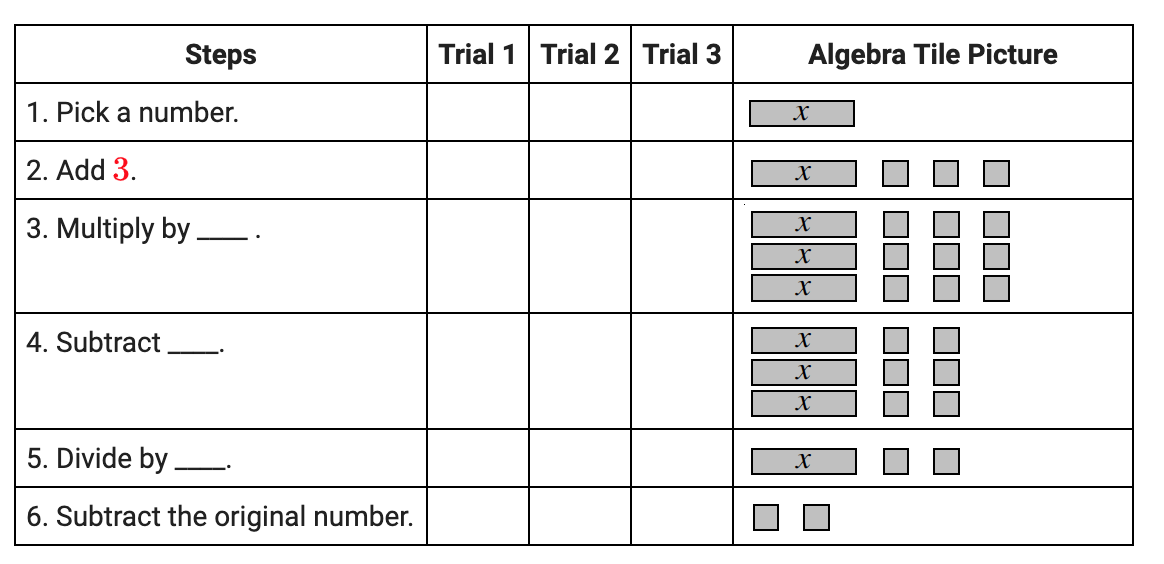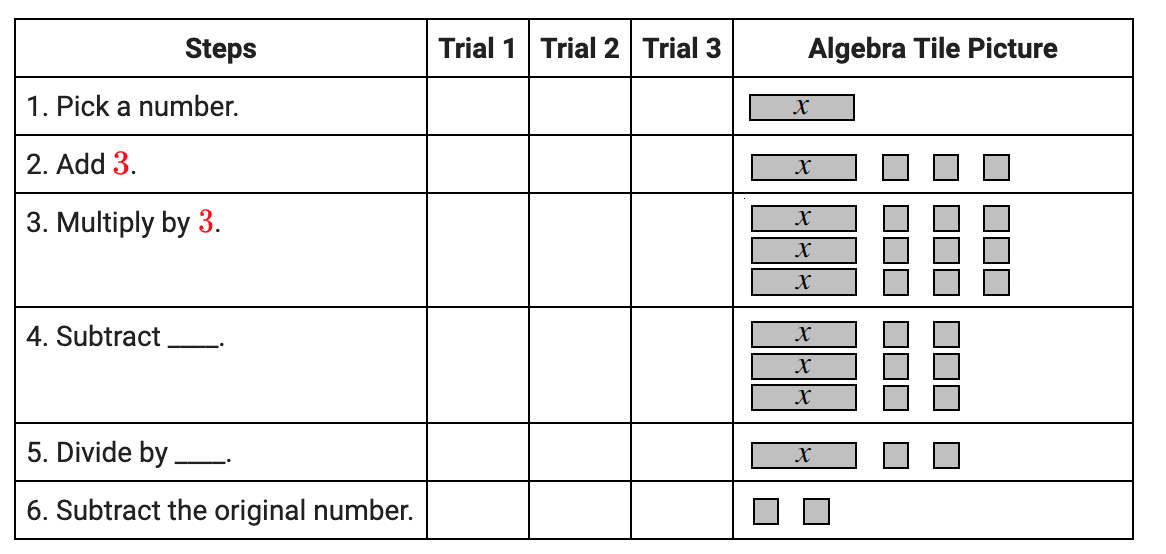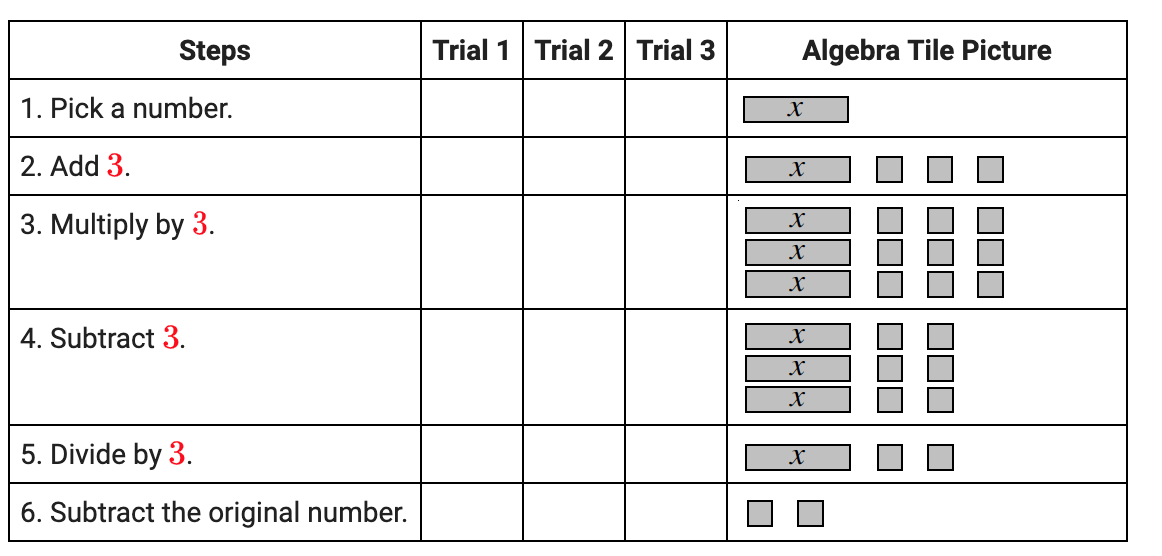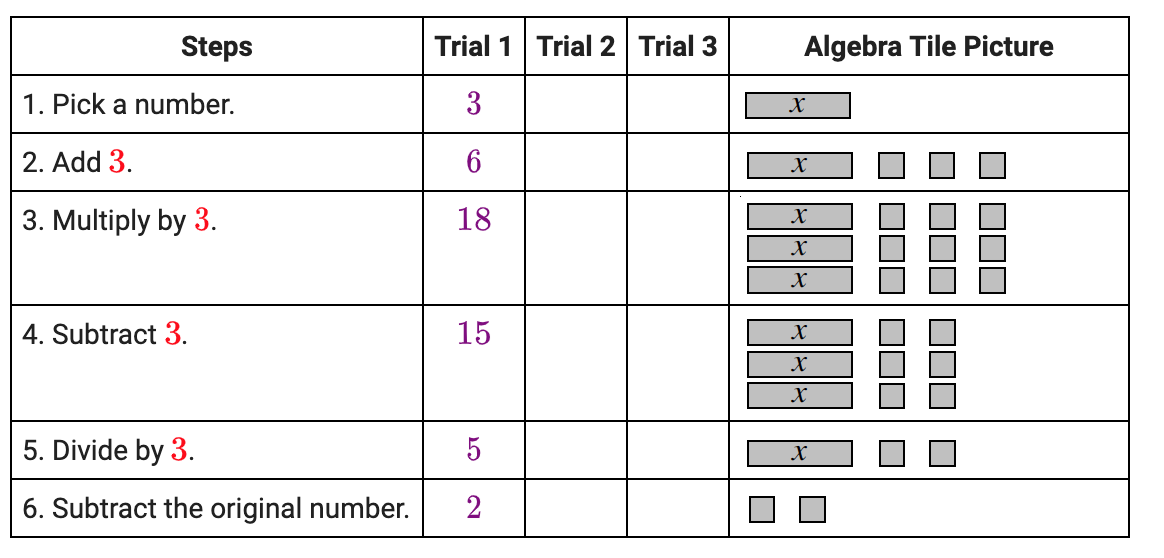### Home > CC1MN > Chapter 9 > Lesson 9.3.1 > Problem9-87

9-87.

Draw the table below on your paper and look carefully at the algebra tiles to fill in each of the steps. Use your own numbers in the trials, again considering fractions, decimals, and zero.

Steps

Trial 1

Trial 2

Trial 3

Algebra Tile Picture

1. Pick a number.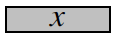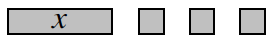3. Multiply by ____.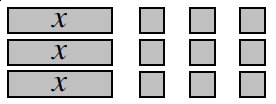4. Subtract ____.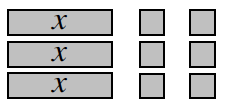5. Divide by ____.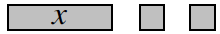6. Subtract the original number.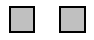Notice how the tiles increase or decrease after each step.

By how much did the algebra tiles increase in Step 2? The third row of the table, first column, now says: 2. Add 3

By how much was that number multiplied in Step 3?
Now complete the rest of the steps using this method. The fourth row of the table, first column, now says: 3. Multiply by, 3.

Substitute in your own numbers for each trial. The fifth row of the table, first column, now says: 4. Subtract, 3. The sixth row of the table, first column, now says: 5. Divide by, 3.

The second column of the table, now says: Trial 1, 3, 6, 18, 15, 5, 2.